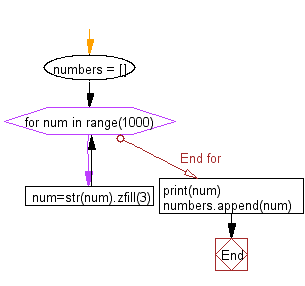﻿ Python: Create the combinations of 3 digit combo - w3resource# Python: Create the combinations of 3 digit combo

## Python Basic - 1: Exercise-5 with Solution

Write a Python program to create the combinations of 3 digit combo.

Sample Solution:

Python Code :

``````numbers = []
for num in range(1000):
num=str(num).zfill(3)
print(num)
numbers.append(num)
``````

Sample Output:

```999
```

Pictorial Presentation:Flowchart:## Visualize Python code execution:

The following tool visualize what the computer is doing step-by-step as it executes the said program:

Python Code Editor :

Have another way to solve this solution? Contribute your code (and comments) through Disqus.

What is the difficulty level of this exercise?

Test your Programming skills with w3resource's quiz.

﻿

## Python: Tips of the Day

What is the difference between Python's list methods append and extend?

append: Appends object at the end.

```x = [1, 2, 3]
x.append([4, 5])
print (x)
```

Output:

```[1, 2, 3, [4, 5]]
```

extend: Extends list by appending elements from the iterable.

```x = [1, 2, 3]
x.extend([4, 5])
print (x)
```

Output:

```[1, 2, 3, 4, 5]
```

Ref: https://bit.ly/2AZ6ZFq